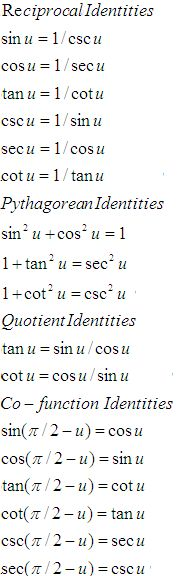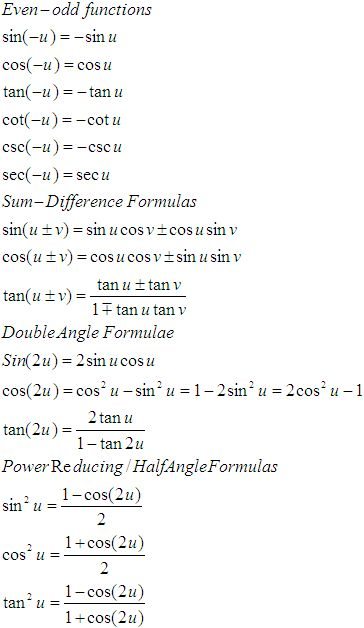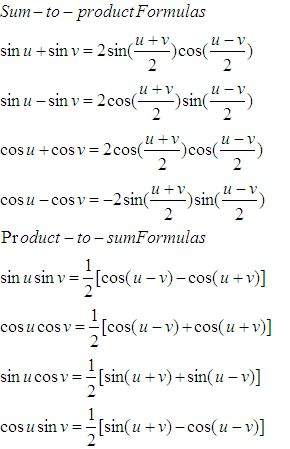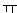Email us to get an instant 20% discount on highly effective K-12 Math & English kwizNET Programs!

#### Online Quiz (WorksheetABCD)

Questions Per Quiz = 2 4 6 8 10

### Geometry8.17 Trignometry Review

 Function 0o 30� 45� 60� 90� sin �0/2 �1/2 �2/2 �3/2 �4/2 cos �4/2 �3/2 �2/2 �1/2 0 tan 0 �3/3 1 �3 undefined sec 1 2�3/3 �2 2 undefined csc undefined 2 �2 2�3/3 1 cot undefined �3 1 �3/3 0

 sin cos tan 0 0 1 0 90 1 0 infinity 180 0 -1 0 270 -1 0 infinityFunctions of angles in all quadrants in terms of those in quadrant 1
 -A 900 � A P/2 � A 1800 � A P � A 2700 � A 3P/2 � A 3600 � A 2P � A sin -sinA cos A �sin A -cosA �sin A cos cos A �sin A -cos A �sin A cos A tan -tan A �cot A � tan A �cot A �tan A csc -csc A sec A � csc A -sec A �csc A sec sec A � csc A -sec A �csc A sec A cot -cot A � tan A � cot A �tan A � cot A

 Q 1: If tan C = 11, find sin C. cos C.Answer: Q 2: The angles of a triangle ABC are in AP and b:c = 31/2:21/2. Find angle A.45 degrees75 degrees60 degrees Q 3: In triangle ABC, a = 50, c = 60, b = 100 degrees, find b.8084.585 Q 4: Find the length of an arc of a circle of radius 10 cm which subtends an angle of 45o at the centre.3/47/45/4 Q 5: In a circle of diamter 40 cm, the length of a chord is 20 cm. Find the length of the minor arc of the chord./35/320/3 Q 6: What is the angle of elevation of the sun when the length of the shadow of a pole is 31/2 times the height of the pole?60o30o90o Q 7: The angle of elevation of the top of a tower which is yet incomplete at a point 120 metres from its base is 45o. How much higher should it be raised so that the elevation at the same point may become 60o?100 metres87.84 metres36.85 metres Q 8: Solve the triangle ABC given that A = 67o, b = 3 cms, c = 2 cms.a = 5 cm, B = 70o19l, C = 39o 41la = 2 cm, B = 73oC = 39o 41la = 2.88 cm, B = 73o19l, C = 39o 41l Question 9: This question is available to subscribers only! Question 10: This question is available to subscribers only!